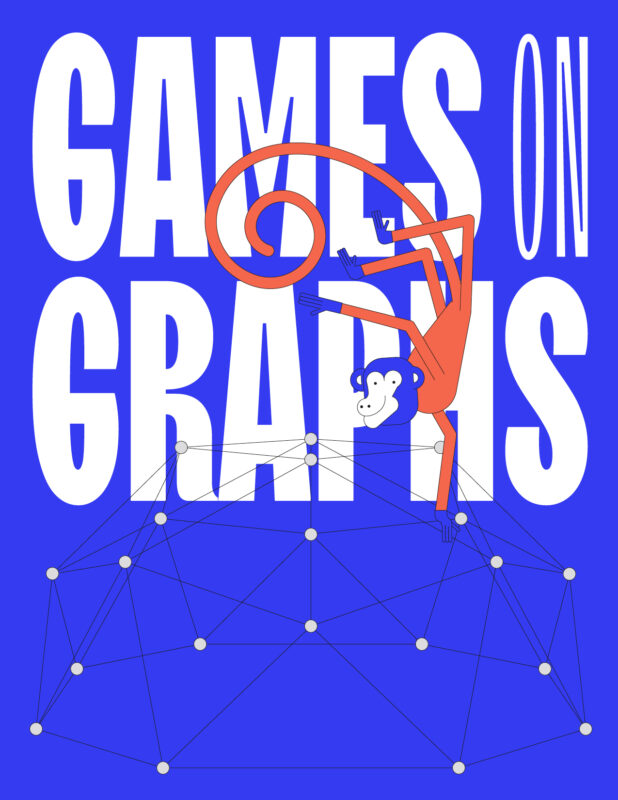# Nathanaël Fijalkow

I am a computer scientist working on program synthesis, games, and automata.

program synthesis

games

automata

## Research topics

### My habilitation, published in Feb 2022, is a good introduction to my research: it's 20 pages and non-technical. Click here

The conception of computer programs is a complicated, costly, and error-prone task. Program synthesis is an ideal where the program is automatically generated from its specification. I am particularly interested in inductive synthesis, where the specification consists of a set of examples.

Reactive synthesis is the special case of program synthesis where the program takes actions over time in a partially controllable environment. I focus on temporal synthesis, where the specification is given by a logical formula in linear temporal logic (LTL) and its extensions.

Games on graphs is at the intersection of several fields: verification (model-checking games such as parity games), logic and model theory (Ehrenfeucht–Fraïssé games), automata theory (emptiness and acceptance games), reinforcement learning (Markov decision processes), and optimisation (mean payoff and discounted games).

Markovian models are stochastic models with memoryless dynamics. The distinction with probabilistic automata is that Markovian models such as Markov decision processes are fully observable.

A dynamical system follows the evolution of a point through repeated applications of a function; the special case of linear dynamical systems is concerned with linear functions, i.e. multiplication by a matrix. Their algorithmic study is deeply intertwined with deep insights from algebraic number theory and geometry. I am particularly interested in invariants for linear dynamical systems, and in related control problems.

The study of probabilistic automata, in particular algorithms for learning and controlling them, has many applications, including program verification, natural language processing, modelling of biological systems, and machine learning.

## Games on GraphsThe Games on Graphs book is online (and free)! It's a collaborative textbook on Games on Graphs: 490 pages, 13 chapters, 17 authors, and even more monkeys.

## Chat TGV

What would happen if Chat TGV could reason, cite, justify?